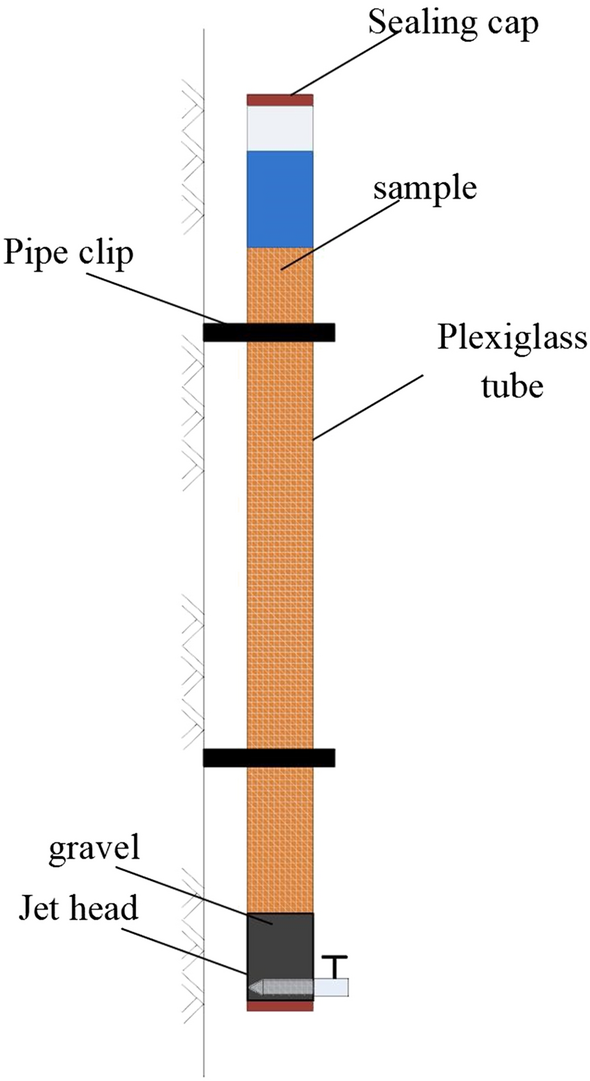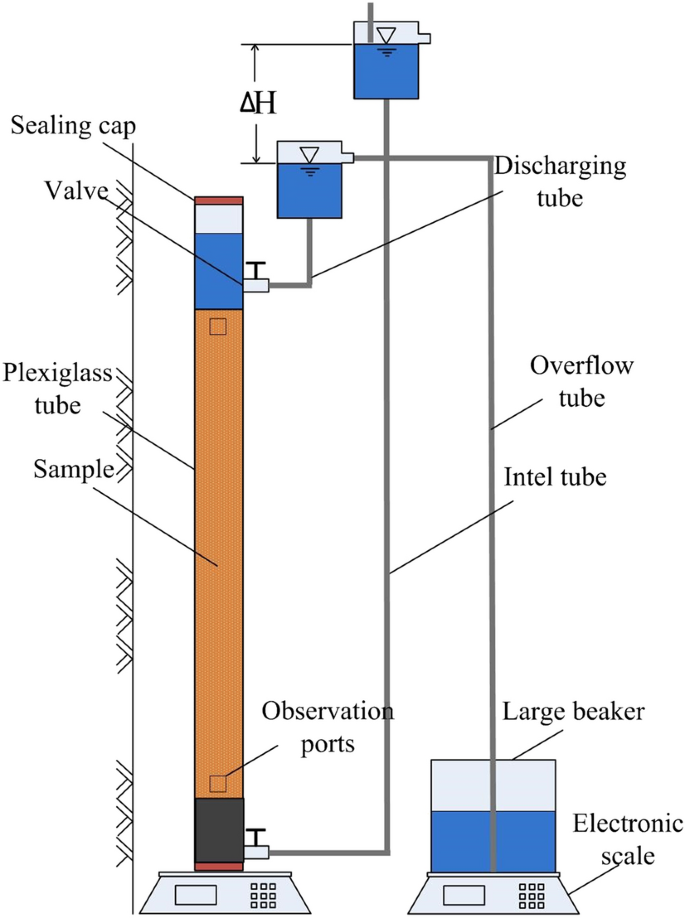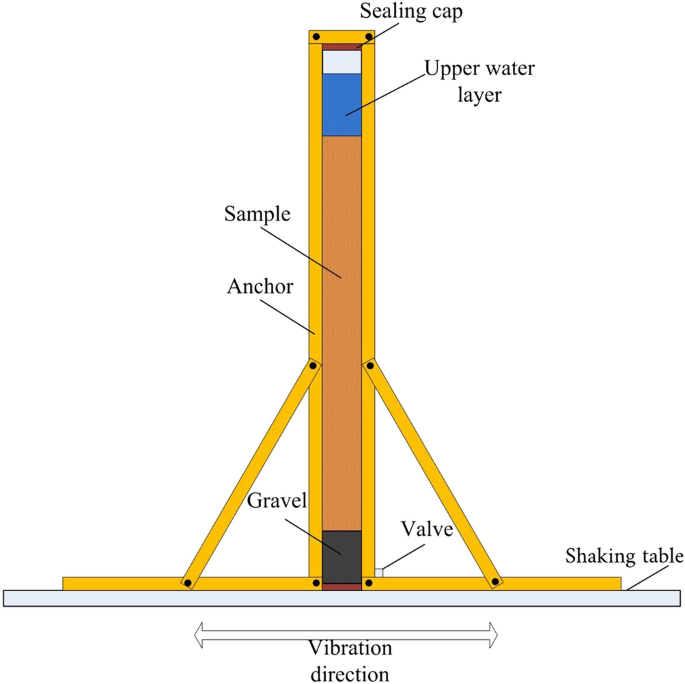# Long-term sustainability of biogas bubbles in sand### Materials

#### Sand

The sand used for this study is source from Nanjing in Jiangsu Province. Conventional tests of specific gravity and particle classification of sand obtained that the specific gravity of sand was 2.68, and the maximum and minimum void ratios of sand were 0.946 and 0.428 after the conversion of corresponding minimum and maximum dry densities directly measured. Figure 1 shows the particle size distribution curve of the sand, and the gradation parameters of the sand are summarized in Table 1.

In Table 1, d10 is the effective diameter, d30 is the medium diameter, d50 is the average grain diameter, and d60 is the constrained diameter, Cu is the non-uniformity coefficient, Cc is the curvature coefficient. It can be seen from Fig. 1 and Table 1 that the content of coarse sand group is more than 50%, without gravel and fine grains, and Cu < 5 and Cc < 1. According to Reference30, it is named as poorly graded sand.

#### Bacteria and denitrifying cultivation

The bacteria strain used was P. stutzeri31 (German Collection of Microorganisms and Cell Cultures [DSMZ]32 5190). It is necessary to note that “5190” is the serial number of the bacteria in the official website of DSMZ. For denitrifying cultivation of the bacteria, the medium was composed of the following components: 0.2 g of magnesium sulfate heptahydrate, 2 g of potassium nitrate, 1 g of dipotassium phosphate, 5 g of sodium citrate dihydrate, and the addition of distilled water a total volume of 1 L.The equation for denitrification of P. stutzeri DSMZ 5,190 is as follows:

$$5{text{C}}_{5} {text{H}}_{7} {text{O}}_{5} {text{COO}}^{ – } + 18{text{NO}}_{3}^{ – } to 30{text{CO}}_{2} + 9{text{N}}_{2} uparrow + 6{text{H}}_{2} {text{O}} + 23{text{OH}}^{ – }$$

In order to verify the stability of nitrogen in the pores of soil, digital camera was used to monitor the nitrogen.

### Methods

#### Hydrostatic condition

The setup for testing of the long-term sustainability of biogas bubbles under hydrostatic conditions is shown in Fig. 2. First, full saturated sand samples were prepared in a plexiglass tube. The height of the sample in the test was 0.8 m. The height of the plexiglass tube was 1.0 m, the outer diameter was 10.5 cm, and the inner diameter was 10 cm. A certain amount of bacterial suspension was added to the bottom of the model, and then sand was poured evenly from the top of the model. The sand sample was compacted once for every 10 cm rise, and the same compaction work was used for each compaction. The sand was below the liquid level all the time. The sample reached a predetermined height, and the top of the sample was tightly closed with a 1 cm-thick piece of liquefied paraffin to avoid evaporation. After denitrification, the height of the top surface of the liquefied paraffin was measured, and the initial degree of saturation of the sample was calculated.Figure 2

The calculation formula is as follows:

$$V_{a} = Delta h times A$$

(1)

$$S_r = (V_{v} – V_{a} – V_{ao} )/V_{v}$$

(2)

where (S_r) is the sample’s degree of saturation, (V_v) is the pore volume, (V_a) is the bacterial gas production, (V_{ao}) is the initial gas content of the sample, (Delta h) is the variation of water thickness on the top surface of the sample, and (A) is the cross-sectional area of the sample.

Second, bubble sustainability was observed. In the first three months, the data were recorded daily; weekly measurements were performed for the rest of the observation period, and the measuring temperature was 20 °C.

#### Hydraulic gradient flow condition

A schematic of the experimental device for the evaluation of biogas bubble stability under different seepage conditions is shown in Fig. 3. On the basis of the hydrostatic condition test, a bucket, water head, and electronic scale were added. The inlet and outlet water tubes and overflow tubes were plastic tubes with diameters of 1–2 cm. The precision of the electronic scale was 0.5 g, and the range was 30 kg. After the preparation of the sample containing biogas bubbles, the plexiglass tube was filled with a bacterial suspension, and the top of the plexiglass tube was sealed with a seal cap. The stability of the biogas bubbles in the soil under different hydraulic gradients was measured by a constant head test.Figure 3

Two types of bubbles were used: accumulated biogas bubbles and dissolved biogas bubbles. Sample saturation could be measured by two methods. In the first method, if biogas bubbles come out in a gas phase, they accumulate at the top of the tube. Then, the volume of this portion could be measured. The reduction in gas volume (Delta V_a) could be measured as well. Thus, sample saturation could be calculated. In the second method, if biogas bubbles come out dissolved from the samples, water would occupy the space vacated by the biogas bubbles. The reduction of biogas volume in this form could be evaluated by measuring the increase in the weight of the sample. The seepage discharge was measured by an electronic scale, and the coefficient of permeability of the soil sample was calculated on the basis of the hydraulic gradient. The equation is

$$Delta V_a = Delta m/rho$$

(3)

where (Delta m) is the change in sample mass and (rho) is the density of water.

Hydraulic gradients of 0.1, 0.2, 0.3, 0.4, and 0.5 were adopted. The test was conducted by increasing the hydraulic gradient gradually. Data were read every 12 h until the degree of saturation was no longer changed for 24 h. The void ratio is calculated by measuring the height of sand sample.

#### Horizontal excitation condition

The setup is schematically illustrated in Fig. 4. The partially saturated sample preparation method is the same as the hydrostatic condition. Under seismic load, if the biogas bubbles overflow in the sample, the upper water layer will drop. Measuring the thickness change of the upper water layer and calculating the volume loss of the bubbles are necessary. Given that volume strain will occur when the sample is under seismic load, the bubble overflow amount is not the only factor that determines the soil degree of saturation. Furthermore, the height of the sample should be measured to obtain the volume strain. Therefore, on the basis of biogas overflow amount and the volume strain of the sample, the degree of saturation can be calculated. In the hydrostatic condition test, the test equipment still needed a small model shaking table, wooden frame, and other equipment. The vertical plexiglass tube was placed on a small shaking table. A wooden block was used to enable the tube and table to vibrate simultaneously and thereby eliminate any possible redundant rocking motion. The cyclic loading was sine waves with 2 Hz. The amplitudes of the sine waves were 0.5, 1.0, 1.5, and 2.0 m/s2. After loading 300 cycles 10 times for each level of load, 10,000 cycles were loaded 10 times. The specimen was loaded with 41,200 cyclic loads. The calculation method for the degree of saturation was consistent with the hydrostatic condition.Figure 4